## Smith Charts Explained

### Why We Need Smith Charts

In the world of RF (Radio Frequency) electronics, normal “bench-top” circuit components cease to operate the way they were designed to.  This means a normal resistor can become a capacitor, a capacitor can become an inductor, and a normal wire can become a distributed network of inductors and capacitors.  This highly non-ideal behavior occurs because, in reality, no true resistor, capacitor, inductor, or wire exists; rather they are all processed and manufactured to operate within a certain frequency range – at frequencies where the real-world effects are quantitatively insignificant.  However, as one approaches RF frequencies, these real-world effects become much more pronounced in cheap components.   Eventually, the frequency of operation can become so high that the transmission line itself – no longer a simple wire – will exhibit significant signal-loss.  But even with lossless transmission lines, it is important in communications to “match impedances,” i.e. attach an antenna whose impedance matches that of the signal source – this maximizes the transmitting-antenna’s power dissipation (and “reflects” back zero power).  Indeed, being able to calculate and measure the impedances of antennas, transmission lines, etc is very important within RF design, which are almost always complex numbers.  Another reason determining load impedances is important is because of the 1:1 mapping between a value of load impedance and a corresponding value of$\Gamma$, the reflection coefficient (a ratio of how much a signal is reflected versus how much a signal is radiated for a given load).

### How To Read a Smith Chart

One way of simplifying the analytical problems communication engineers typically face is by using a Smith Chart.  Smith Charts provide a graphical representation of the impedance of any load – whether that load be an antenna or simply an open-circuited transmission line, such as a coax cable.  Because these impedances may very well be complex in nature, a Smith Chart is designed such that each point on it represents both the real and imaginary parts of the load’s impedance.   To begin, observe the basic “format” of any Smith Chart:

What is seen here is the generic, normalized Smith Chart.  Each point on the chart represents an impedance, and the numbers marked on the chart represent different coefficients needed to multiply by the original load value  (from which the chart was normalized from – If this is confusing, don’t worry about it – the “original load value” will almost always be known).  The chart consists of yellow circles and red arcs.  The yellow circles represent contours of where the Real part of the impedance magnitude is the same, e.g. for any point along the yellow circle marked “2.0,” the real part of the impedance is: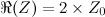where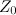is the impedance the chart was normalized from.

Similarly, the red arcs represent contours where the Imaginary part of the impedance magnitude is the same, e.g. for any point along the red arc marked “0.5,” the imaginary part of the impedance is: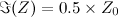This is very handy for displaying complex impedance values.  For instance, notice the following Smith Chart:

What is the impedance for the load represented on the Smith Chart by the blue dot?  This is easy to determine, because:

1. The blue dot is along the yellow circle marked “2.0,” so the real part of the impedance must be: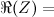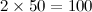2. The blue dot is also along the red arc marked “0.5,” so the imaginary part of the impedance must be: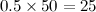So, from this, we have determined that the impedance of the “load” represented by the blue dot is: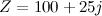By using this method, it is simple to find the impedance represented by any point on the Smith Chart!

### Useful Smith Chart Relations

As mentioned before, a Smith Chart is really just a 1:1 mapping between a value of load impedance and a value of$\Gamma$, the reflection coefficient of a load.  The reflection coefficient is defined as:$\Gamma =\frac{V_{reflected}}{V_{incident}} = \frac{V_{refl}}{V_{inc}}$

The reflection coefficient is a very important metric.  For antennas, a reflection coefficient expresses how much signal voltage is used in exciting the antenna and how much signal voltage is reflected back to the source.  For an ideal antenna,$\Gamma$ would be zero – corresponding to$Z = Z_0 \Omega$, which is the origin of the Smith Chart!  In fact, simple relations exist between$Z$ and$\Gamma$: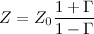and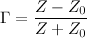Useful Notes

Simple observations allow for a more “intuitive” approach when using Smith Charts.  Note the following:

• The straight red line in the center is an “arc” representing all points where the imaginary part of the impedance is zero
• The furthest-left point on the straight red line represents where the impedance is zero (or, a short circuit), and the furthest-right point on the straight red line represents where the impedance is$\infty$ (or, an open circuit)
• The very top point of the Smith Chart is where the impedance is +$j$
• For this reason, the top half of the Smith Chart represents inductive loads
• The very bottom point of the Smith Chart is where the impedance is –$j$
• Similarly, the bottom half of the Smith Chart represents capacitive loads

____

Safa Khamis